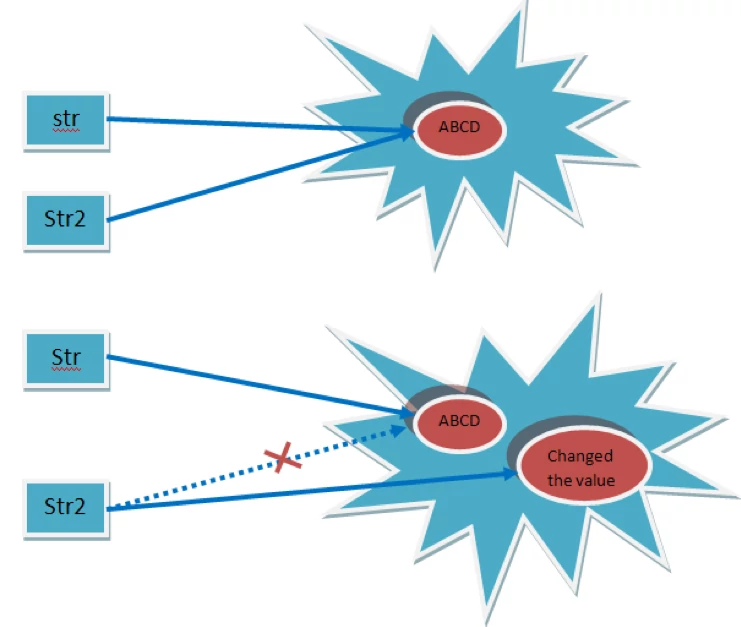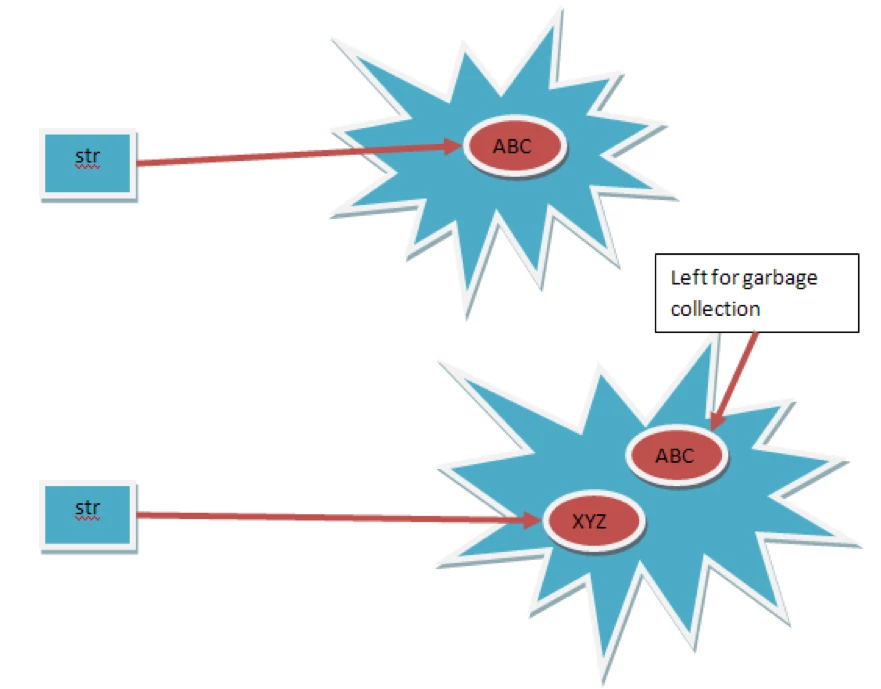In Java, strings are objects. Just like other objects, you can create an instance of a String with the new keyword, as follows:

``````String s = new String("abc");
``````

This line of code creates a new object of class String, assigns it to the reference variable s and initialized it. So far, String objects seem just like other objects.

## Constructors

``````String()
String(byte[] bytes)
String(byte[] ascii, int hibyte)
String(byte[] bytes, int offset, int length)
String(byte[] ascii, int hibyte, int offset, int count)
String(byte[] bytes, String charsetName)
String(char[] value)	String(String original)
String(byte[] bytes, int offset, int length, String charsetName)

``````

We can write it in this way also:-

``````String s = "abc";
``````

Now we create a new reference s2 to s

``````String s2 = s;
``````

So far this is behaving same as other classes but with next step the expected result is different, Let first see what it he result.

``````public class TestObjectReference {
private String value;

public TestObjectReference(String value) {
this.value = value;
}
public String getValue() {
return value;
}
public void setValue(String value) {
this.value = value;
}
}
``````
``````public class StringTest {

public static void main(String[] args){
//Working with any Object class
TestObjectReference reference = new TestObjectReference("abcd");
TestObjectReference reference2 = reference;

reference2.setValue("Changed the value");
System.out.println(reference.getValue());

System.out.println("***********Let us try now with String Class **************");

String str = "ABCD";
String str2 = str;

str2 = "Changed the value";

System.out.println(str);
}
}
``````
``````Changed the value
***********Let us try now with String Class **************
ABCD
``````

We do not get the expected output, so where is the problem, where are we making the mistakes.

The problem is String are immutable objects, means whenever we make any change to the value a new String object is created.Let us consider another case:-

``````String str = "ABC";
str = "XYZ";
``````

In this case too we haveSo now we consider a situation in which we have too many String operations then in that case we may run out of memory as every time you modify a String a new memory location is allocated.

To solve this problem we have another Class provided for String manipulation, i.e. the StringBuffer class.

## Important Methods in the String Class

The following methods are some of the more commonly used methods in the String class.

### public char charAt(int index)

This method returns the character located at the String’s specified index. Remember, String indexes are zero-based—for example

``````String x = "airplane";
System.out.println( x.charAt(2) ); // output is 'r'
``````

### public String concat(String s)

This method returns a String with the value of the String passed in to the method appended to the end of the String used to invoke the method.

``````String x = "taxi";
System.out.println( x.concat(" cab") ); // output is "taxi cab"
``````

# public boolean equalsIgnoreCase(String s)

This method returns a boolean value (true or false) depending on whether the value of the String in the argument is the same as the value of the String used to invoke the method. This method will return true even when characters in the String objects being compared have differing cases.

``````String x = "Exit";
System.out.println( x.equalsIgnoreCase("EXIT")); // output is "true"
System.out.println( x.equalsIgnoreCase("tixe")); // output is "false"
``````

# public int length()

This method returns the length of the String used to invoke the method

``````String x = "01234567";
System.out.println( x.length() ); // returns "8"
``````

# public String replace(char old, char new)

This method returns a String whose value is that of the String used to invoke the method, updated so that any occurrence of the char in the first argument is replaced by the char in the second argument

``````String x = "oxoxoxox";
System.out.println( x.replace('x', 'X') ); // output is // "oXoXoXoX"
``````

# public String substring(int begin) / public String substring(int begin, int end)

The substring() method is used to return a part (or substring) of the String used to invoke the method.

``````String x = "0123456789";// as if by magic, the value
//of each char
//is the same as its index!
System.out.println( x.substring(5) ); // output is "56789"
System.out.println( x.substring(5, 8)); // output is "567"
``````

When we are specifying the second argument ‘end index’, it will always take the value till the (end -1) just as in above example, substring (5, 8) will retrieve only “567” as output.

# public String toLowerCase()

This method returns a String whose value is the String used to invoke the method, but with any uppercase characters converted to lowercase

``````String x = "A New Moon";
System.out.println( x.toLowerCase() ); // output is "a new moon"
``````

### public String toString()

This method returns the value of the String used to invoke the method. What? Why would you need such a seemingly “do nothing” method? All objects in Java must have a toString() method, which typically returns a String that in some meaningful way describes the object in question.

``````String x = "big surprise";
System.out.println( x.toString() ); // output is obviously the same.
``````

### public String toUpperCase()

``````String x = "A New Moon";
System.out.println( x.toUpperCase() ); // output is "A NEW MOON"
``````

### public String trim()

This method returns a String whose value is the String used to invoke the method, but with any leading or trailing blank spaces removed.

``````String x = " hi ";
System.out.println( x + "x" ); // result is " hi x"
System.out.println( x.trim() + "x"); // result is "hix"
``````